# Properties of Rational Numbers

To know the properties of rational numbers, we will consider here the general properties of integers which include associative, commutative and closure properties. Rational numbers are the numbers which can be represented in the form of p/q, where q is not equal to 0. Basically, the rational numbers are the integers which can be represented in the number line. Let us go through all the properties here.

## Properties of rational numbers

Rational means anything which is completely logical whereas irrational means anything which is unpredictable and illogical in nature. The word rational has evolved from the word ratio. In general, rational numbers are those numbers that can be expressed in the form of p/q, in which both p and q are integers and q≠0. We can denote these numbers by Q.

### Closure property

For two rational numbers say x and y the results of addition, subtraction and multiplication operations give a rational number. We can say that rational numbers are closed under addition, subtraction and multiplication. For example: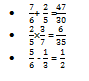Do you know why division is not under closure property?

Division is not under closure property because division by zero is not defined. We can also say that except ‘0’ all numbers are closed under division. For example:

Division is not under closure property because division by zero is not defined. We can also say that except ‘0’ all numbers are closed under division. For example: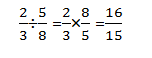### Commutative law

For rational numbers, addition and multiplication are commutative.

Commutative law of addition: a+b = b+a

Commutative law of multiplication: a×b = b×a

For example: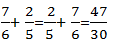Subtraction is not commutative property i. e. a-b ≠ b-a. This can be understood clearly with the following example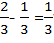whereas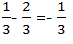Division is also not commutative i.e. a/b ≠ b/a as,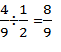whereas,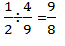### Associative law

Suppose x, y and z are rational then for addition: x+(y+z)=(x+y)+z

For multiplication: x(yz)=(xy)z.

Some important properties that should be remembered are:

• 0 is an additive identity and 1 is a multiplicative identity for rational numbers.
• For a rational number  x/y is  the additive inverse of